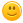# How do divide whole numbers by fractions? ex.-6 divided by 2/3

237 views
asked Jan 27, 2016 in Math

I am sure i am going to learn this in school, but i really want to be "ahead" of the class like the smart ones. I know how to divide whole numbers by UNIT fractions but i don't know how to divide whole numbers by REGULAR fractions Please help me!!answered Jan 27, 2016 by (65,660 points)
It is really simple if you know the trick. The trick is understanding that whatever you do equally to the top and bottom of a fraction does not change it.

Example:  5/7  I can multiply the top and bottom by 3 and nothing changes

15/21 = 5/7

So you use the same concept to clear the fractions. In your example you have

-6 / (2/3)

Multiply the bottom by 3 and top by 3 to make the bottom part a whole number.

-6 * 3 / (2/3)*3 = -18 / 2

Now simplify: -18/2 = -9

In more advanced math, like algebra, you will use this concept all the time, but instead of top / bottom, you can do anything as long as you do it to both sides of the equals sign. Very powerful concept that will take  you far in math.
commented Jan 27, 2016 by Lou Lou
thanku